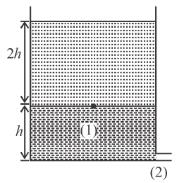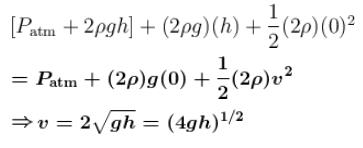Courses

# Fluid Dynamics NAT Level - 2

## 10 Questions MCQ Test Topic wise Tests for IIT JAM Physics | Fluid Dynamics NAT Level - 2

Description
This mock test of Fluid Dynamics NAT Level - 2 for Physics helps you for every Physics entrance exam. This contains 10 Multiple Choice Questions for Physics Fluid Dynamics NAT Level - 2 (mcq) to study with solutions a complete question bank. The solved questions answers in this Fluid Dynamics NAT Level - 2 quiz give you a good mix of easy questions and tough questions. Physics students definitely take this Fluid Dynamics NAT Level - 2 exercise for a better result in the exam. You can find other Fluid Dynamics NAT Level - 2 extra questions, long questions & short questions for Physics on EduRev as well by searching above.
*Answer can only contain numeric values
QUESTION: 1

### A cube with a mass m = 20g wettable by water floats on the surface of water. Each face of the cube a is 30 cm long. What is the distance between the lower face on the cube and the surface of the water (in mm)?   [S.T of water = 70 dyn/cm, g = 10 m/sec2, assume angle of contact with water is zero]

Solution:

Downward force = Buoyant force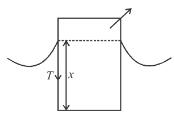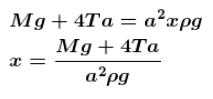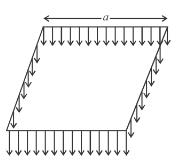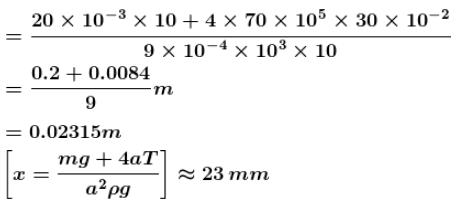*Answer can only contain numeric values
QUESTION: 2

### If the container filled with liquid gets accelerated horizontally or vertically, pressure in liquid gets changed. In case of horizontally accelerated liquid (ax), the free surface has the slope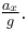In case of vertically accelerated liquid (ay) for calculation of pressure, effective g is used. A closed box with horizontal base 6m by 6m and a height 2m is filled half with liquid. It is given a constant horizontal acceleration and vertical downward acceleration g/2. The angle (in degrees) of the free surface with the horizontal is equal to :

Solution: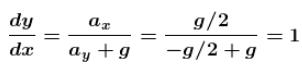...(effective g will be g – a = g/2)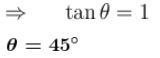*Answer can only contain numeric values
QUESTION: 3

### A Siphon tube is discharging a liquid of specific gravity 0.9 from a reservoir as shown in the figure. The pressure at the highest point B is given by k × 104 Pa. Find the value of k.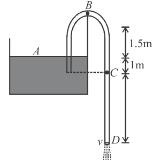Solution: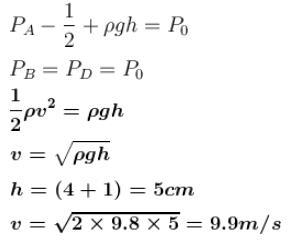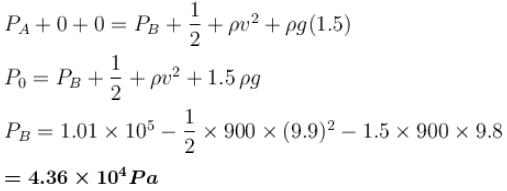*Answer can only contain numeric values
QUESTION: 4

A cylindrical tank 1 m in radius rests on a platforms 5 m high. Initially the tank is filled with water to a height of 5m. A plug whose area is 10–4 m2 is removed from an orifice on the side of the tank at the bottom. Calculate time taken (in seconds) to empty the tank to half its original values.

Solution: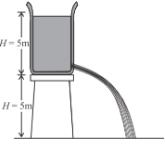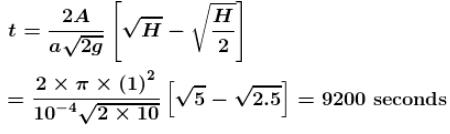*Answer can only contain numeric values
QUESTION: 5

The U-tube acts as a water siphon. The bend in the tube in 1m above the water surface. The water issues from the bottom of the siphon as a free jet at atmospheric pressure. Determine the minimum absolute pressure of the water in the bend is k ×104 N/m2. Find the value of k.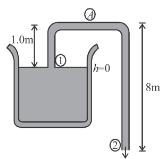Solution:

Applying Bernoulli equation between points (1) and (2)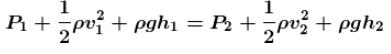Since, area of reservoir >> area of pipe.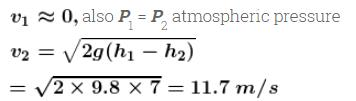The minimum pressure in the bend will be at A. Therefore applying
Bernoulli’s equation between (1) and A.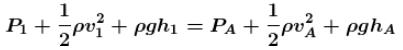again v1 ≈ 0 and from conservation of mass VA = V2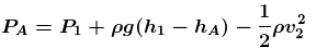on putting the value, we have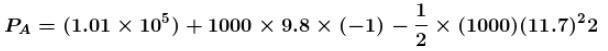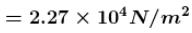*Answer can only contain numeric values
QUESTION: 6

Water filled in an empty tank of area 10A through a tap of cross sectional area A. The speed of water flowing out of tap is given by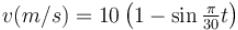where t is in seconds. The height of water level from the bottom of the tank at t = 15 sec will be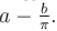Find the value of (a + b).

Solution:

Volume of water filled in tank in t = 15 sec.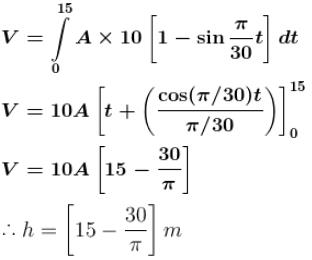*Answer can only contain numeric values
QUESTION: 7

An open tank 10m long and 2m deep is filled up to filled 1.5m  height with oil of specific gravity 0.82. The tank is uniformly accelerated along its length from rest to a speed of 20m/sec  horizontally. The shortest time (in seconds) in which the speed may be attained without spilling any oil is : [g = 10m/sec2]

Solution: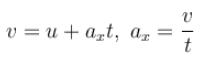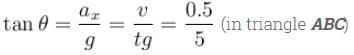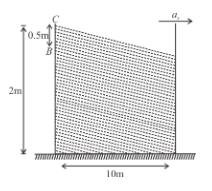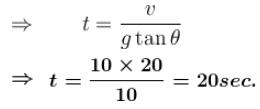*Answer can only contain numeric values
QUESTION: 8

When jet of liquid strikes a fixed or moving surfaces, it exerts thrust on it due to rate of change of momentum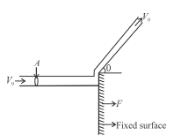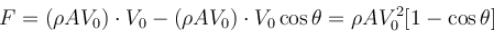If surface is free and starts moving due to thrust of liquid, then at any instant, the above equation gets modified based on relative change of momentum with respect to surface. Let any instant the velocity of surface is u, then above equation becomes :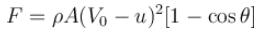Based on above concept, in the below given figure, if the cart is frictionless and free to move in horizontal direction. Then velocity (in m/s) of cart at t = 10 sec is equal to

Solution: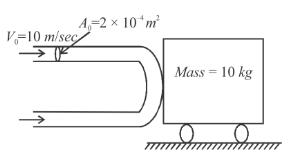Given cross-section area of jet = 2 × 10–4m2 velocity of jet V0 = 10 m/sec., density of liquid = 1000 kg/m3,
Mass of cart = M = 10 kg.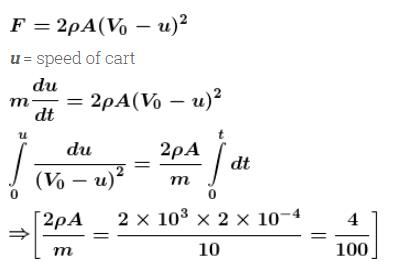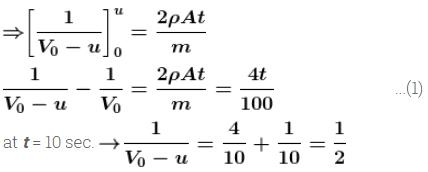V0 – u = 2
u = 8m/sec

*Answer can only contain numeric values
QUESTION: 9

Length of horizontal arm of a uniform cross-section U-tube is l = 21cm and ends of both of the vertical arms are open to surrounding of pressure 10500 N/m2. A liquid of density is poured into the tube such that liquid just fills the horizontal part of the tube. Now one of the open ends is sealed and the tube is then rotated about a vertical axis passing through the other vertical arm with angular velocity  If length of each vertical arm be a = 6cm. Calculate the length of air column in the sealed arm in cm. [g = 10 m/sec2]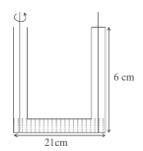Solution:

Due to rotation, Let the shift of liquid is x cm.
Let cross-section area of tube = A
In the right limb for compressed air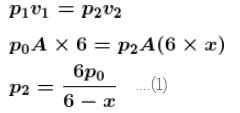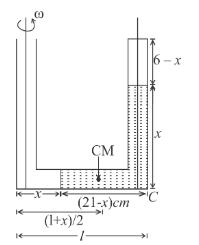Force at the corner C of right limb climb due to liquid above.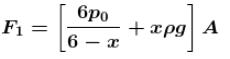Mass of the liquid in horizontal arm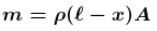It is rotated about left limb, then centripetal force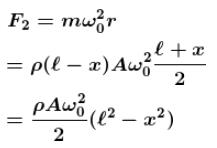But F1 = F2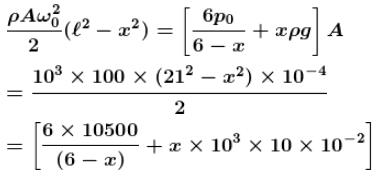On solving x = 1 cm
then length of air column = 6 – 1 = 5 cm

*Answer can only contain numeric values
QUESTION: 10

The velocity of the liquid coming out of a small hole of a vessel containing two different liquids of densities 2ρ  and ρ as shown in figure is given by (ngh)1/2.  Find the value of n.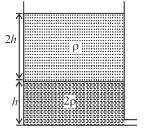Solution:

Pressure at (1) :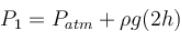Applying Bernoulli’s theorem between points (1) and (2)